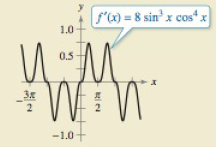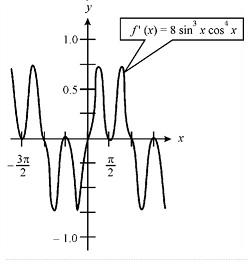Chapter 8.3, Problem 68E

Chapter
Section
Textbook Problem

# HOW DO YOU SEE IT? Use the graph of f’ shown inlbe figmto answer the following.(a) Using the interval shown in the graph, approximate the value (s) of x where f is maximum. Explain.sUsing the interval shown in the graph, approximate the value (s) of x where f is minimum. Explain.

(a)

To determine

To calculate:

The value of x where the function f is maximum in the following graph.Explanation

Given:

The following graph

Formula used:

f'(x)=0

Calculation:

Consider the following function y=f(x)

Then the derivative of this function with respect to x will be:

dydx=f'(x)

Now for a function if f'(x)=0 then the point will be maxima or minima

(b)

To determine

To calculate:

The value of x where f is minimum in the following graph### Still sussing out bartleby?

Check out a sample textbook solution.

See a sample solution

#### The Solution to Your Study Problems

Bartleby provides explanations to thousands of textbook problems written by our experts, many with advanced degrees!

Get Started

#### Solve the equations in Exercises 126. 4xx313x4x31x31=0

Finite Mathematics and Applied Calculus (MindTap Course List)

#### In problems 45-62, perform the indicated operations and simplify. 58.

Mathematical Applications for the Management, Life, and Social Sciences

#### Divide: 15x65x4

Elementary Technical Mathematics

#### Solve 578=234x.

Mathematics For Machine Technology